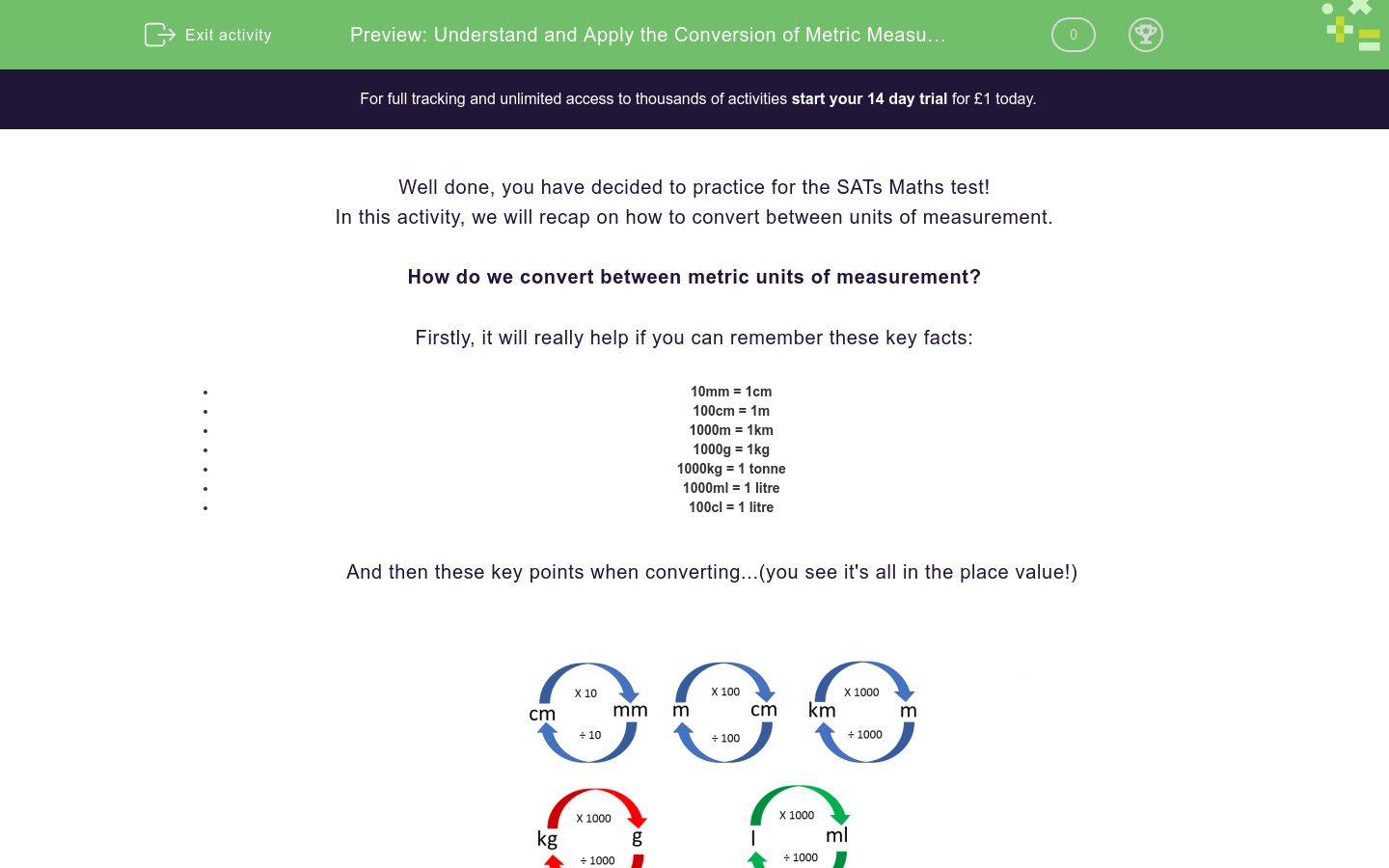# Understand and Apply the Conversion of Metric Measurement Units

In this worksheet, students will be asked to convert between units of measurement. The student will be asked to convert between metric units of measure.Key stage:  KS 2

Curriculum topic:   Exam-style Questions: SATs Maths

Curriculum subtopic:   Exam-Style Questions: Decimal Conversion

Difficulty level:#### Worksheet Overview

Well done, you have decided to practice for the SATs Maths test!

In this activity, we will recap on how to convert between units of measurement.

How do we convert between metric units of measurement?

Firstly, it will really help if you can remember these key facts:

• 10mm = 1cm
• 100cm = 1m
• 1000m = 1km
• 1000g = 1kg
• 1000kg = 1 tonne
• 1000ml = 1 litre
• 100cl = 1 litre​

And then these key points when converting...(you see it's all in the place value!)Now, time to have a go at some practice questions!

### What is EdPlace?

We're your National Curriculum aligned online education content provider helping each child succeed in English, maths and science from year 1 to GCSE. With an EdPlace account you’ll be able to track and measure progress, helping each child achieve their best. We build confidence and attainment by personalising each child’s learning at a level that suits them.

Get started